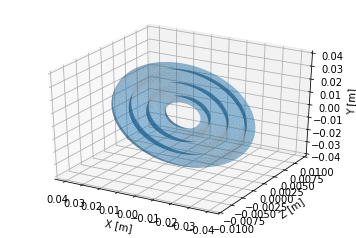# Parametric Surfaces - All About¶

This document describes how parametric surfaces are implemented in OSCARS.

A parametric surface in OSCARS is represented by a python class. This class must contain the variables u and v, start and stop points ustart, ustop, vstart, vstop, and the number of points in each dimension nu, nv. Later in this notebook we develop a custom surface. First we start with surfaces in OSCARS.

In :
# matplotlib plots inline
%matplotlib inline

# Import the OSCARS SR module
import oscars.sr

# Import OSCARS 3D tools (matplotlib)
from oscars.plots3d_mpl import *

# Import OSCARS parametric surfaces
from oscars.parametric_surfaces import *

OSCARS v2.1.8 - Open Source Code for Advanced Radiation Simulation
Brookhaven National Laboratory, Upton NY, USA
http://oscars.bnl.gov
oscars@bnl.gov

In :
# Plot the rectangle
rectangle = PSRectangle(L=0.01, W=0.01, nu=51, nv=51)
plot_surface(rectangle)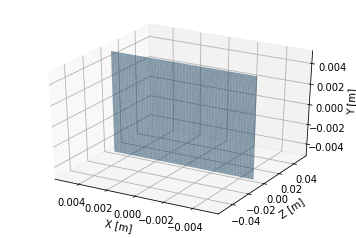In :
# Plot the sphere
sphere = PSSphere(R=0.010, nu=51, nv=51)
plot_surface(sphere)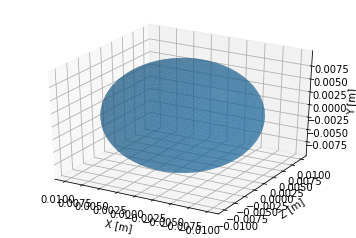In :
# Plot the cylinder
cylinder = PSCylinder(R=0.010, L=0.1, nu=51, nv=51)
plot_surface(cylinder)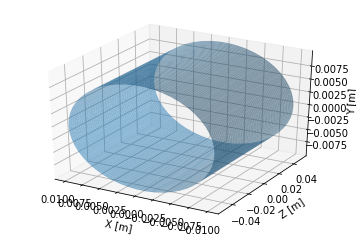In :
# Plot the torus
torus = PSTorus(R=0.010, r=0.005, nu=51, nv=51)
plot_surface(torus)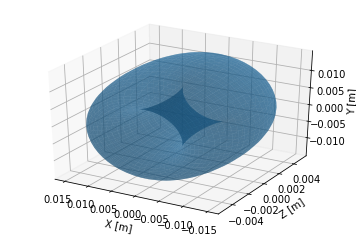# Eample - Custom Parametric Surface in OSCARS¶

The example that follows shows how to create a custom parametric surface. The parametric surface is created in a simple python class. OSCARS requires the class to have the following defined:

Functions: position(u, v), normal(u, v) which each return a 3-element list [x, y, z]

Integers: nu, nv

Floats: ustart, ustop, vstart, vstop

At the moment you must provide the normal. You can use the following recipe for the components of the normal:

In the following, let x = x(u, v) = position(u, v), and so on

$$N_x = \frac{\partial y}{\partial u}\frac{\partial z}{\partial v} - \frac{\partial z}{\partial u}\frac{\partial y}{\partial v}\\ N_y = \frac{\partial z}{\partial u}\frac{\partial x}{\partial v} - \frac{\partial x}{\partial u}\frac{\partial z}{\partial v}\\ N_y = \frac{\partial x}{\partial u}\frac{\partial y}{\partial v} - \frac{\partial y}{\partial u}\frac{\partial x}{\partial v}$$

You need to decide if you want to invert this or not since it's not clear which is the front and back side. It is also a good idea to normalize this.

The position in this example is given by the following: $$x = u \sin(v)\\ y = u \cos(v)\\ z = A \sin( 2 \pi u / \lambda )$$

which gives a normal of: $$N_x = 2 A \pi u \cos(2 \pi u / \lambda) \sin(v) / \lambda\\ N_y = 2 A \pi u \cos(2 \pi u / \lambda) \cos(v) / \lambda\\ N_z = -u$$ and in this example I did have to invert the normal vector

In :
from math import sin, cos, pi, sqrt, exp
class PSWavyDisk:
"""A Parametric surface - wavy disk with a hole in the center"""

# This shape specific parameters
R1 = 0.001
R2 = 0.005
A = 0.001
Lambda = 0.01

# Required for all PS shapes
# Start, stop, and number of points for the u and v parameters
# All PSShapes must have these defined
ustart = R1
ustop  = R2
vstart = 0
vstop  = 2 * pi
nu = 21
nv = 21

def __init__ (self, R1=0.001, R2=0.005, nu=21, nv=21):
self.R1 = R1
self.R2 = R2
self.ustart = R1
self.ustop  = R2
self.nu = nu
self.vstart = 0
self.vstop = 2.*pi
self.nv = nv

def position (self, u, v):
"""Return the position in 3D at this u and v"""

x = u * sin(v)
y = u * cos(v)
z = self.A * sin( 2. * pi * u / self.Lambda )

return [x, y, z]

def normal (self, u, v):
"""Return a unit normal in 3D at this u and v position"""

xn = -2. * self.A * pi * u * cos(2. * pi * u / self.Lambda) * sin(v) / self.Lambda
yn = -2. * self.A * pi * u * cos(2. * pi * u / self.Lambda) * cos(v) / self.Lambda
zn = u

mag = sqrt(xn*xn + yn*yn + zn*zn)

return [xn / mag, yn / mag, zn / mag]

In :
# Create a parametric shape and plot it
wavydisk = PSWavyDisk(R1=0.01, R2=0.04, nu=51, nv=101)
plot_surface(wavydisk, zlim=[-0.01, 0.01])